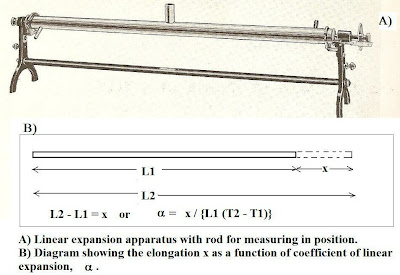## Tuesday, June 7, 2011

### Basic Physics (Thermodynamics) - Pt. 16Thermal expansion, the expansion of metals, objects as a result of temperature difference, is another critical practical application of thermodynamics. As we know from engineering, roads, bridges as well as rails are constructed to take into account expansion. The simplest (relatively) is the case for linear expansion, that is epxansion in one dimension only, though one can also consider expansion of an entire volume, V. We will look in this blog at the basics of measuring the linear expansion, say of a metal rod, then go on to briefly generalize from there to volumes before looking at some problems.

For the experiment, such as we do at Harrison College, the apparatus used is shown. The test rod initial length (L1)is first carefully measured before being inserted in place. (A steam generator equipped with steam jacket is usually used to generate the heat for expansion, though some labs without it simply use Bunsen burners set to very low heat). Four readings are generally taken, first when at the point of the micrometer screw gauge with the rod still at room temperature, call it T1. The heating is then begun and conducted for ~ 5 minutes, or until a temperature of 100C (212F) is attained. At this point, 4 more readings of the expanded rod length (L2) are taken and averaged. Time permitting, the experiment is repeated at least three more times after all components (e.g. steam jacket, rod) have cooled. The procedure can also be repeated for rods of other metals.

The primary calculation is the increase or elongation in length, x = (L2 - L1), given by the difference in micrometer readings for each rod. (The schematic showing what is physically taking place is depicted in (B) of the diagram) To calculate the coefficient of linear expansion, one uses x and the temperature difference recorded, (T2 - T1) to obtain:

alpha = x / {L1 (T2 - T1)}

The units are given in: cm/cm C or C^-1 or m/ m C. Thus, the coefficient alpha is the fractional change (L2 - L1/ L1) per degree change in temperature.

The preceding can be generalized to the case of volumes (taking each dimension to be an expanding LENGTH, but extended to three dimensions, for L, W and H) by allowing a hypothetical volume coefficient of expansion, BETA = 3 (alpha), given:

V = LWH (length x width x height)

Then, if we have (T + delta T) we get (V + delta V)

and

(V + delta V) = (L + delta L) (W + delta W) (H + delta H)

where:

delta L = (alpha)L (delta T) or (L2 - L1)= alpha L1 (T2 - T1)

and similarly,

delta W = (alpha) W (delta T)

delta H = (alpha) H (delta T)

On substituting:

(V + delta V) =(alpha)L (delta T)+ (alpha) W (delta T)+(alpha) H (delta T)

Or: (V + delta V) = LWH [1 + alpha (delta T)]^3

whence:

delta V = V[1 + 3 (alpha)delta T + 3 (alpha*delta T)^2 + (alpha*delta T)^3)]

Since the higher order terms are negligible for most simple experiments, we get:

(delta V)/ V = 3 (alpha)delta T

and BETA (coeff. of volume expansion) = 1/V [(delta V)/(delta T)] = 3 (alpha)

{Note: The industrious reader should be able to show by similar working that a coefficient for area expansion - say of a metal sheet- would be: 2 alpha (delta T))

Sample Problems:

1) An aluminum bar of 1.00000 m length has a coefficient of linear expansion alpha = 0.000022 /C. If the rod is initially at a room temperature of 20 C and is then heated to 100 C, find the new length.

The amount of linear expansion x = alpha {L1 (T2 - T1)}

alpha = 0.000022 /C and (T2 - T1) = (100C - 20C) = 80C

then: x = (0.000022 /C) [1m/ 80C] = 2.75 x 10^-6 m = 2.75 x 10^-3 mm

Then: L2 = L1 + x = 1000 mm + 2.75 x 10^-3 mm = 1000.00275 mm

2) Between 15C and 100C water has a coefficient of volume expansion of BETA = 0.00037/C. If 1 liter of water is heated from 20C (room temp.) to 75C, find the expanded volume.

BETA = 0.00037/C = 1/V [(delta V)/(delta T)] = 3 (alpha)

(delta T) = (75 C - 20 C) = 55C and V = 1L = 1000 cm^3

Then: delta V= (1000 cm^3)(0.00037/C) (55C) = 20.35 cm^3

then the new volume = V + delta V = 1000 cm^3 + 20.35 cm^3 = 1020 cm^3

Problems:

1) A vertical steel antenna tower is 200 m high. Calculate the change in height of the tower that takes place when the temperature changes from -20 C on a winter day to 30 C on a summer day.

2) A metal rod is 200.00 cm long at 0C and 200.18 cm long at 60C. What is its coefficient of linear expansion?

3) A metal vessel has a volume of 500.00 cm^3 at 0 C. If the coefficient of linear expansion is 0.000011 /C, what is the volume at 50 C?

4) A round hole of 6.000 cm in diameter at 0 C is cut in a sheet of brass(alpha = 0.000018 /C). Find the new diameter of the hole at 50 C.